Examples

Chapter 13 Class 8 Introduction to Graphs
Serial order wise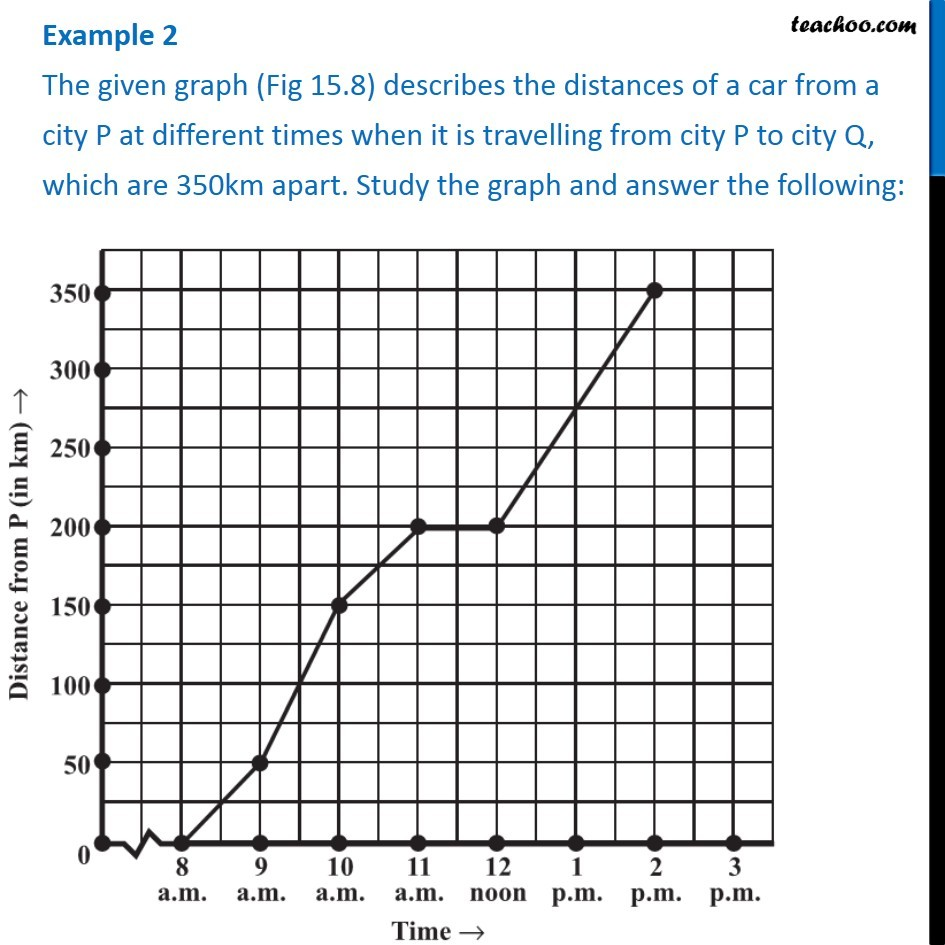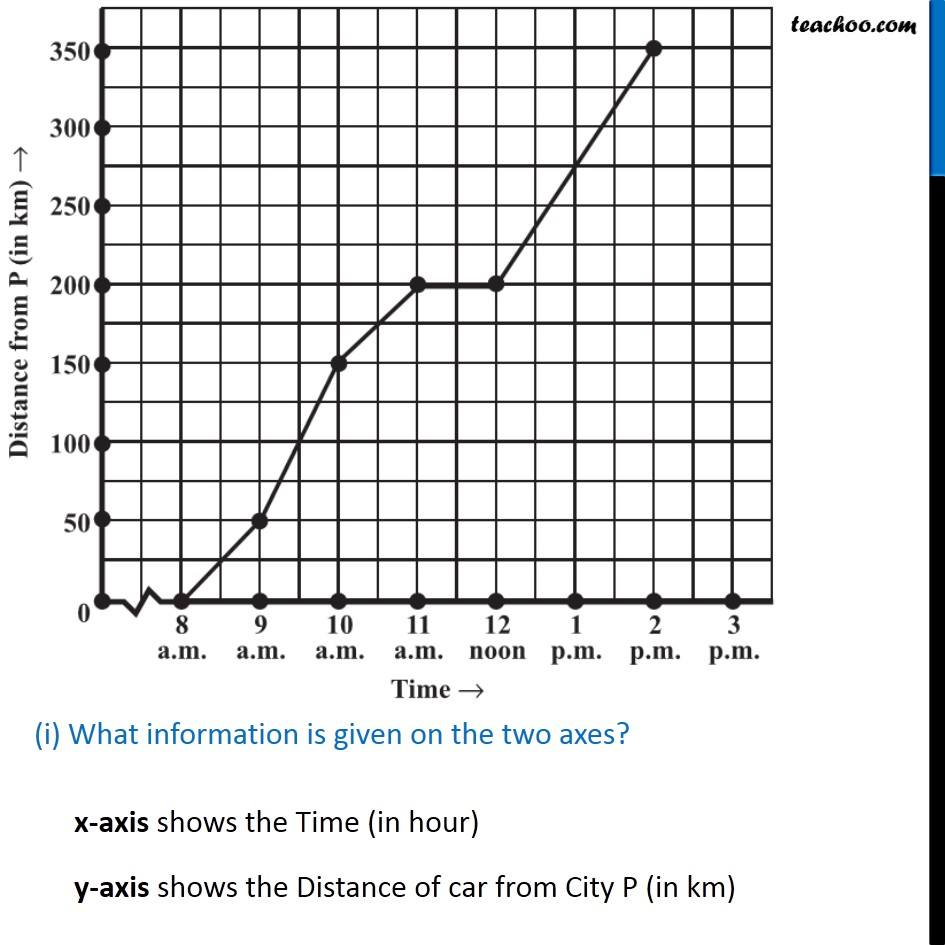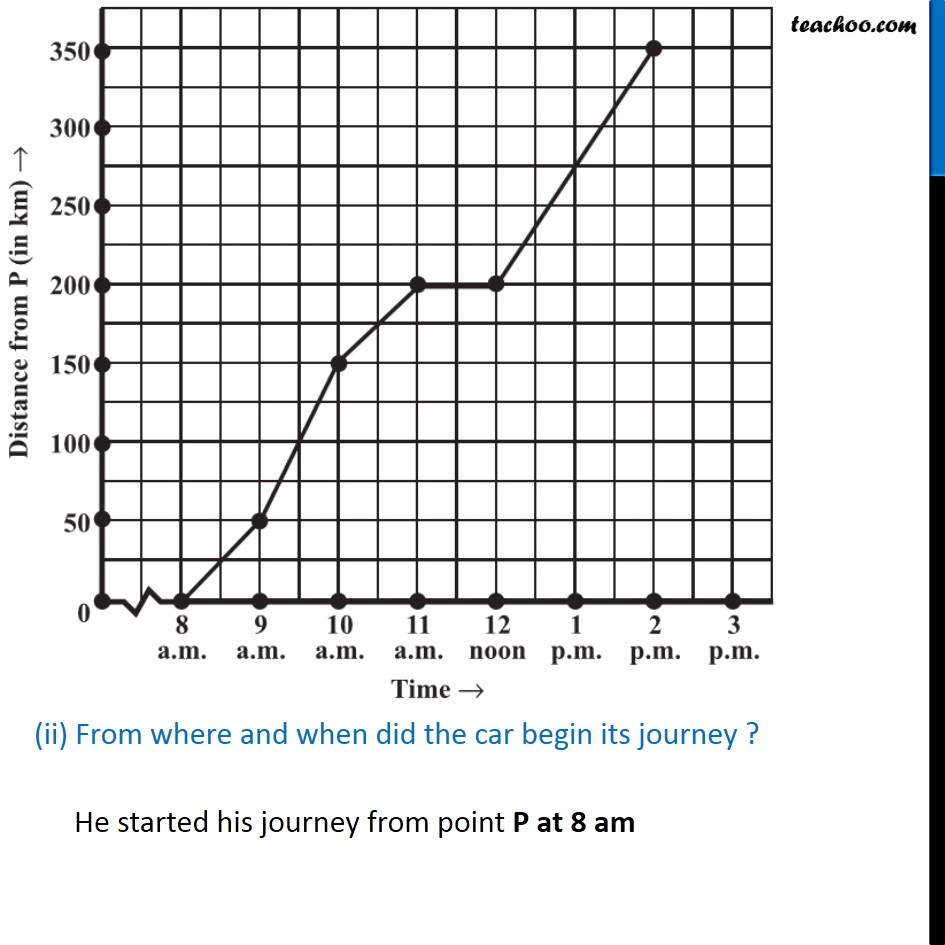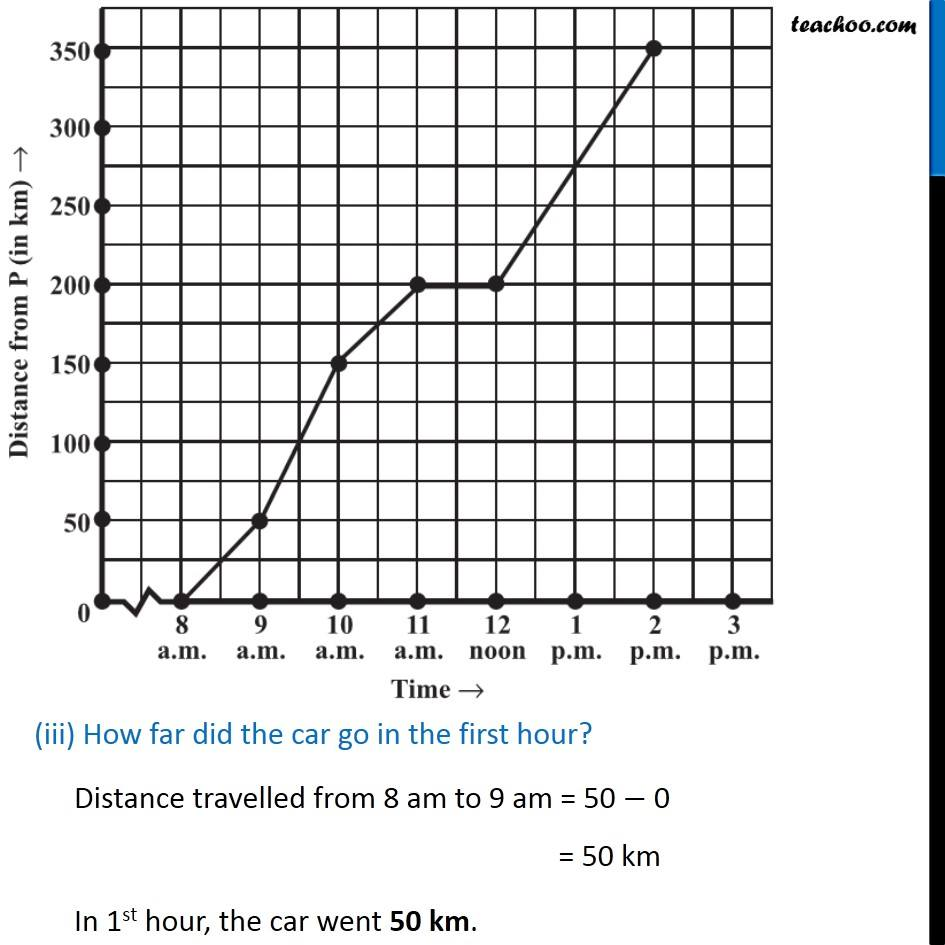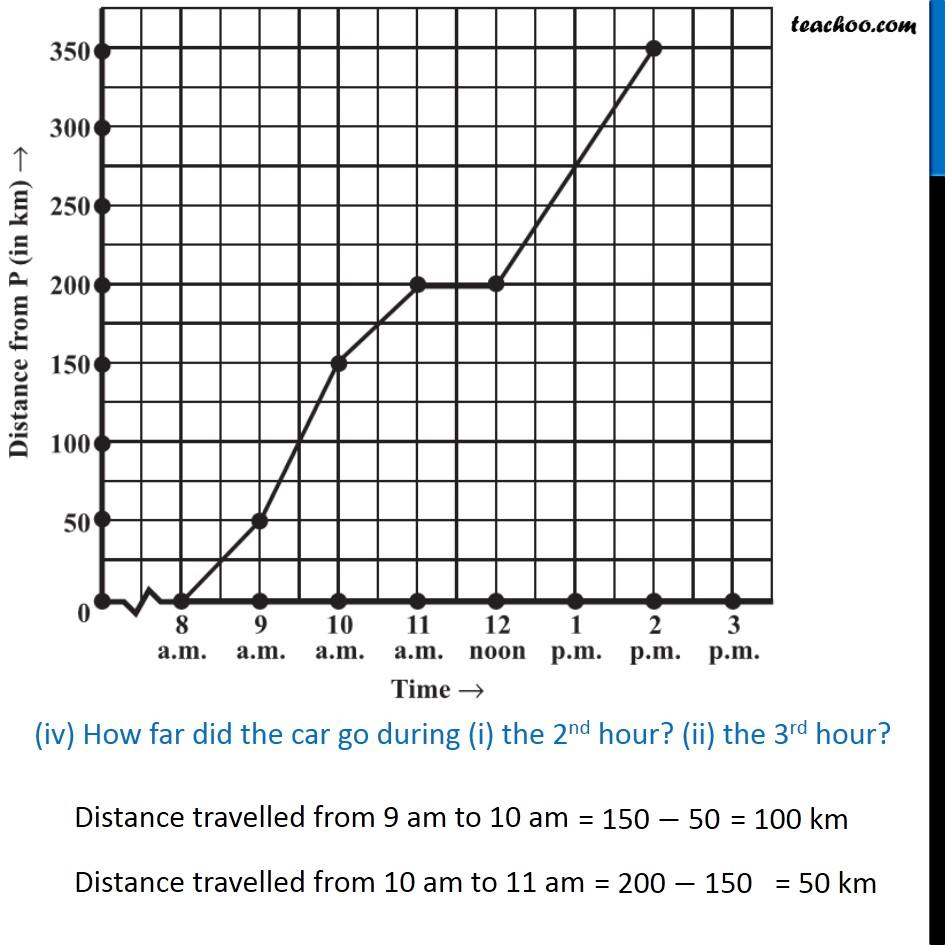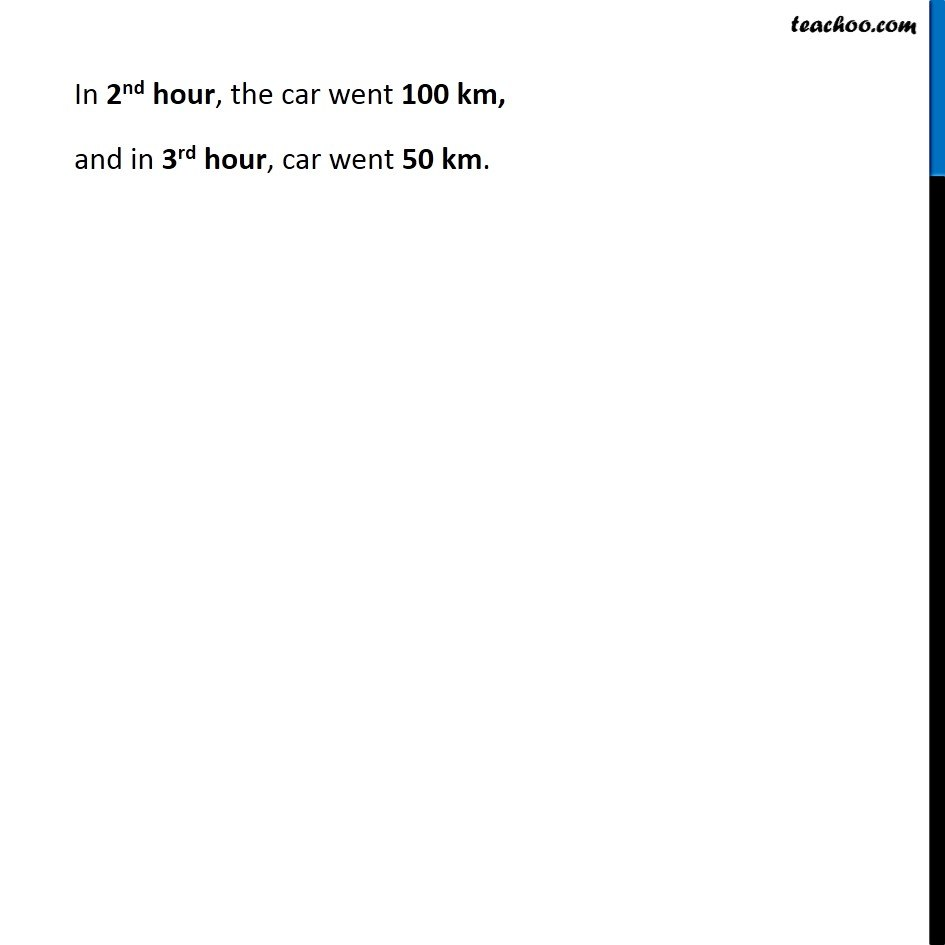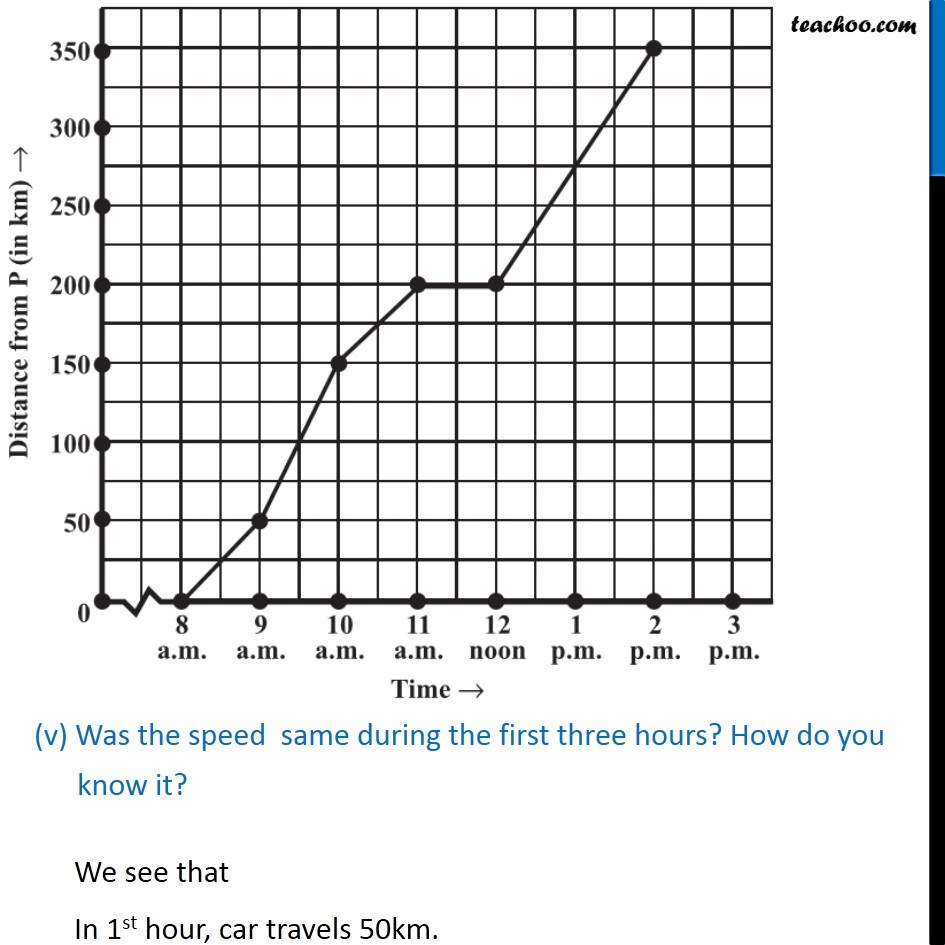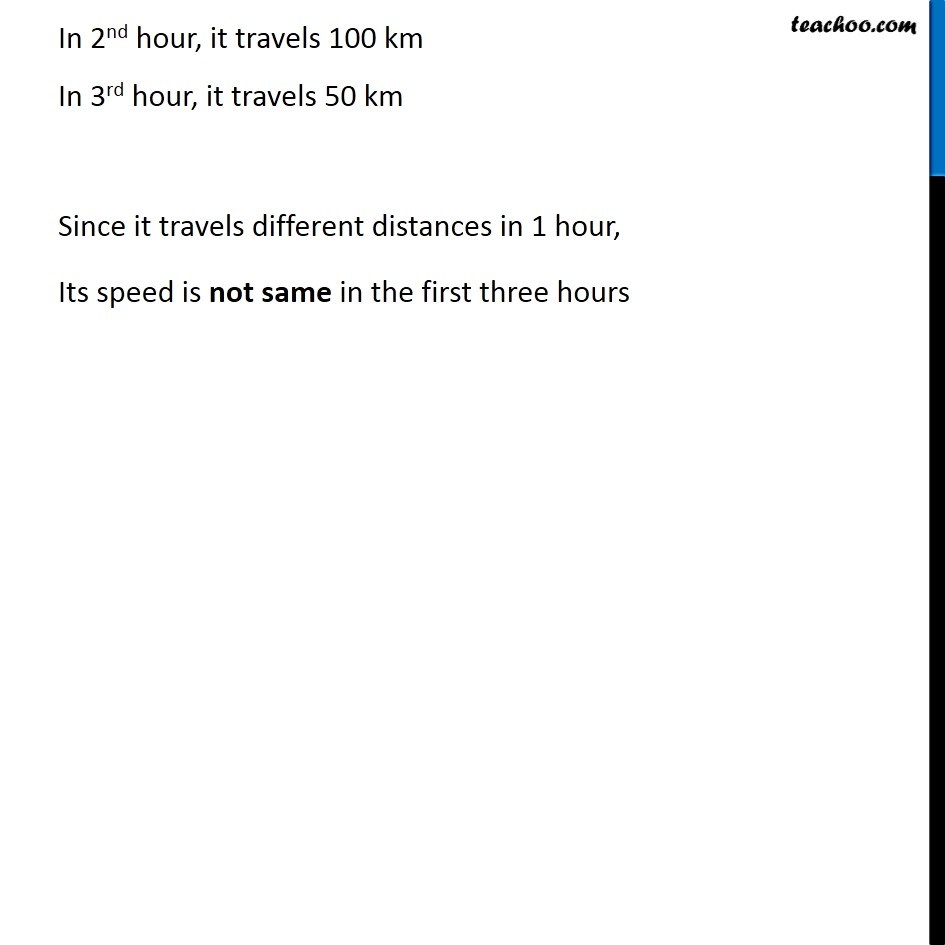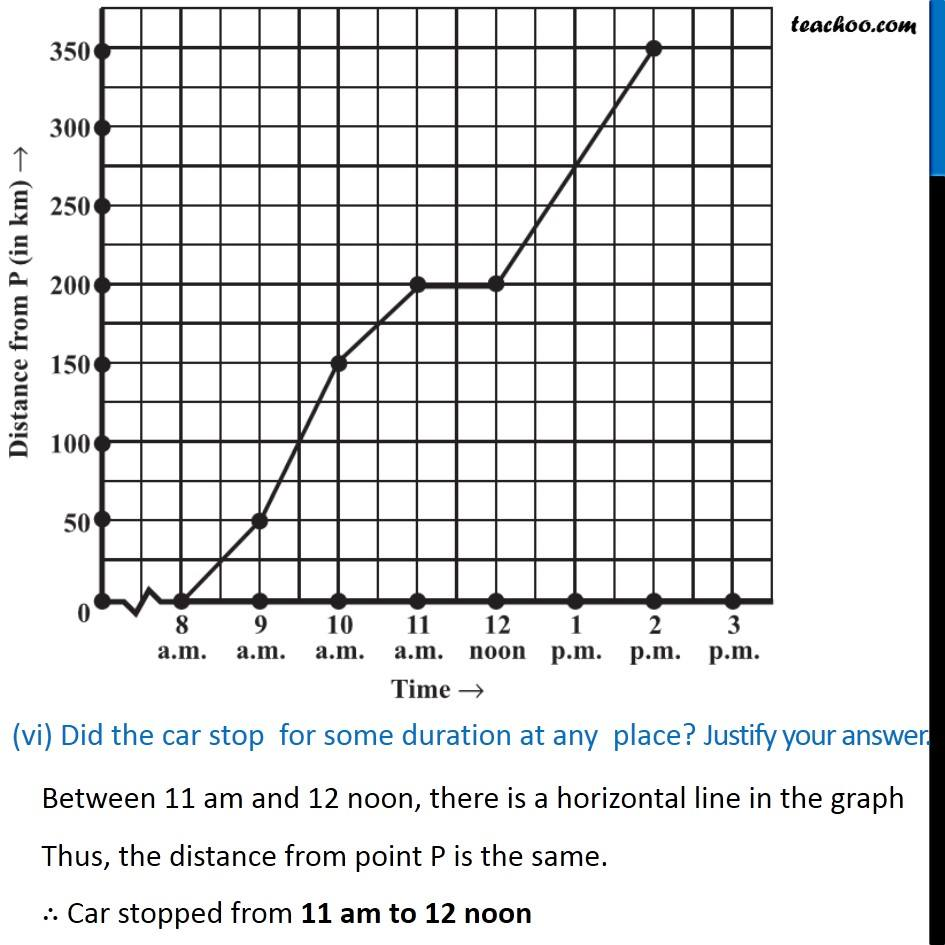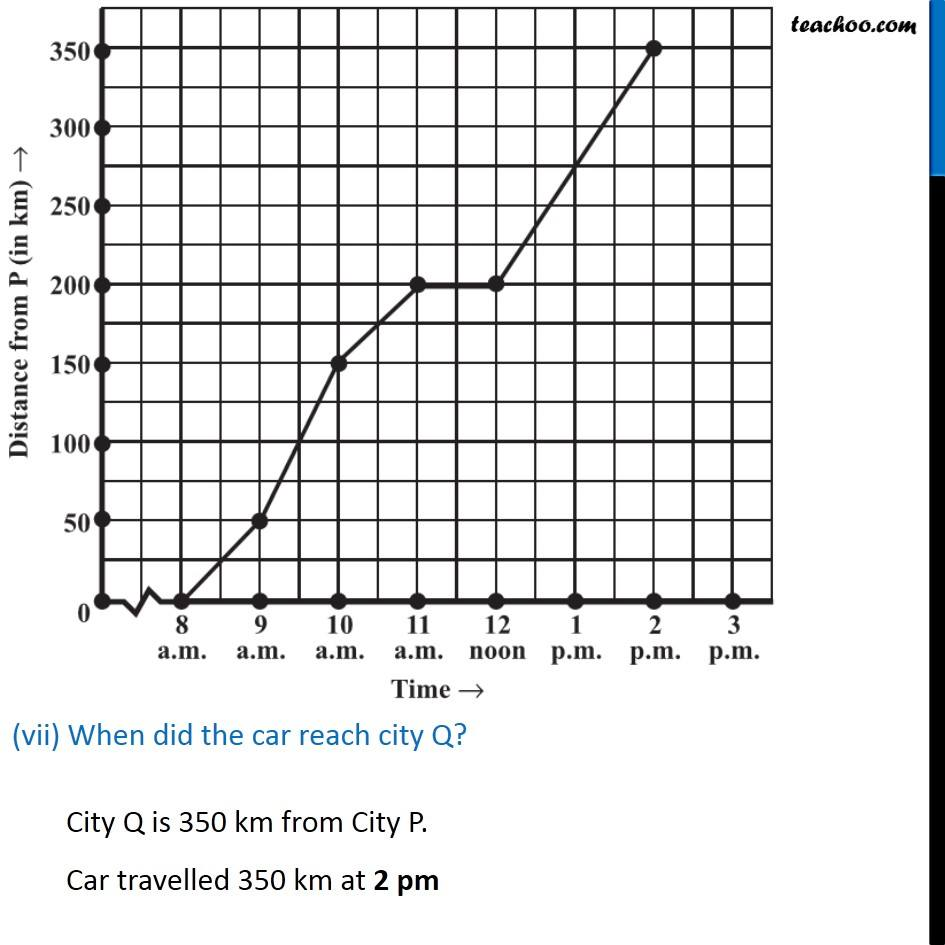Learn in your speed, with individual attention - Teachoo Maths 1-on-1 Class

### Transcript

Example 2 The given graph (Fig 15.8) describes the distances of a car from a city P at different times when it is travelling from city P to city Q, which are 350km apart. Study the graph and answer the following: (i) What information is given on the two axes? x-axis shows the Time (in hour) y-axis shows the Distance of car from City P (in km) (ii) From where and when did the car begin its journey ? He started his journey from point P at 8 am (iii) How far did the car go in the first hour? Distance travelled from 8 am to 9 am = 50 − 0 = 50 km In 1st hour, the car went 50 km. (iv) How far did the car go during (i) the 2nd hour? (ii) the 3rd hour? Distance travelled from 9 am to 10 am Distance travelled from 10 am to 11 am In 2nd hour, the car went 100 km, and in 3rd hour, car went 50 km. (v) Was the speed same during the first three hours? How do you know it? We see that In 1st hour, car travels 50km. In 2nd hour, it travels 100 km In 3rd hour, it travels 50 km Since it travels different distances in 1 hour, Its speed is not same in the first three hours (vi) Did the car stop for some duration at any place? Justify your answer. Between 11 am and 12 noon, there is a horizontal line in the graph Thus, the distance from point P is the same. ∴ Car stopped from 11 am to 12 noon (vii) When did the car reach city Q? City Q is 350 km from City P. Car travelled 350 km at 2 pm